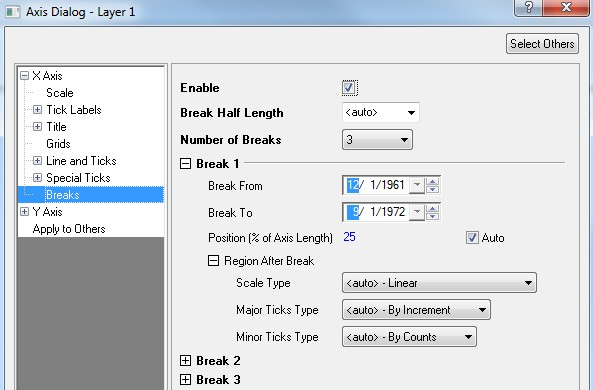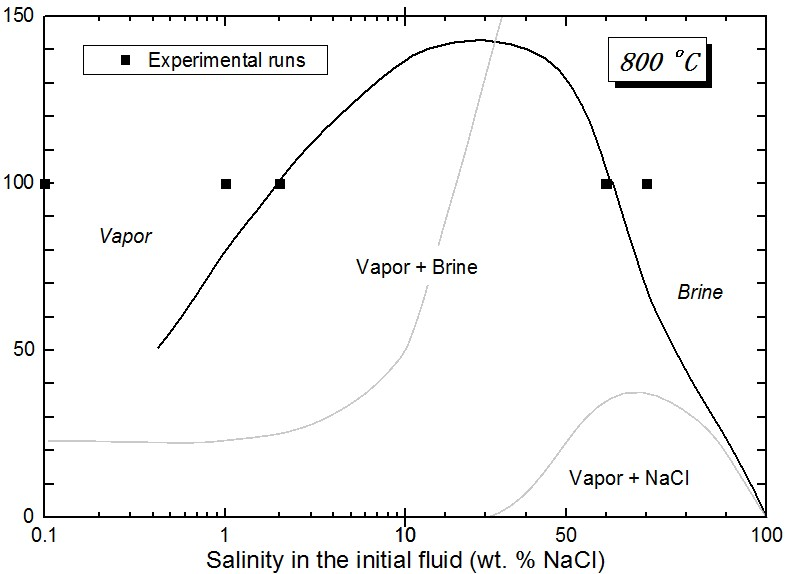# Sample OPJ to download to try: Multi breaks and Other Axis Related.opj

ORG-298ORG-7708, ORG-7762)## Multiple Axis BreaksHow to:

1. Double click the axis you want to add breaks on to open Axis Dialog.
2. In Breaks node in left panel, choose the Number of Breaks you want to put on the axis (up to 7 breaks each axis). They are uniformly distributed by default.
3. Set break symbol size by Break Half Length.
4. Modify each break's detailed setting, include Break From/To, Position, etc..
5. You can also use Labtalk command to enable the axis breaks.
ExampleDescription
layer.x.breaks.enable = 1;1 to turn on axis breaks on x axis, 0 to turn it off.
layer.x.breaks.count = 2;It means Number of Breaks = 2.
layer.x.break#.from = 5;

# could be numerical value. layer.x.break1.from=5 means Region From of the first break is 5.

layer.x.break#.to = 10;# could be numerical value. layer.x.break1.to=10 means Region To of the first break is 10.

## Axis Break without GapHow to:If set break Region From and Region To to be the same value, there will be no gap on the graph. User can use this method to change axis scale.

## Useful Axis Break Properties

Can use LabTalk command to modify the size of break gap. Both X/Y axis break would be effected by layer.x.breaks.gap

ExampleDescription
layer.x.breaks.gap = 100;When value is bigger than 30, means percent of one percent of the axis length. In this case, total width 1% of axis length.
layer.x.breaks.gap = 15;Value between 1-30 is used to expressed in 1/10 of major tick length. 15 means 1.5 times tick length.
layer.x.breaks.gap = 0;No gap
layer.x.breaks.gap = -120;When value is negative, means unit is in 1/10 of point size. -120 means the gap will have 12 point size.
layer.x.breaks.gap = 0/0;Missing value means follow @AB
layer.x.breaks.size= 0;Controls break symbol size. Setting to 0 will show no Break symbol on axis.
layer.x.breaks.enable=1Enable axis breaks
layer.x.breaks.count=2Number of Breaks =2
layer.x.break1.from=151st break's From value
layer.x.break1.to=201st break's To value